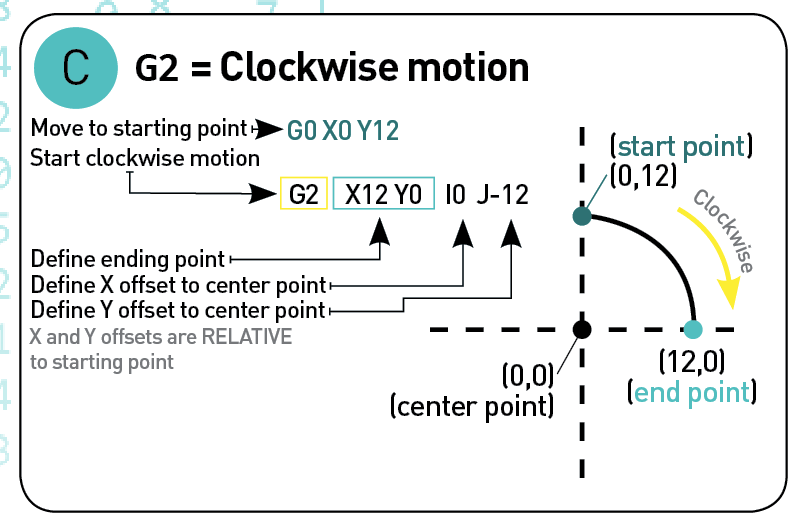## G2 G-code

G2 means "move while activating the tool", in a clockwise arc motion.

This command is equivalent to G1, except the motion is rotational instead of linear.

G2's evil twin is G3, which is exactly the same, except it is counter-clockwise instead of clockwise.

### Format

The command is used as such :

````G2 X10 Y20 I30 J40 F100`
```

Which means : move to X position 10, Y position 20, while maintaining a constant distance from the point of relative coordinates X 30 and Y 40, at a speed of 100 millimeters/minute

### Parameters

Parameter Usage Example
X Move to this position in the X axis G2 X10 I10
Y Move to this position in the Y axis G2 Y10 I10
Z Move to this position in the Z axis G2 Z10 I10
I The point in X space from the current X position to maintain a constant distance from G2 X10 I10
J The point in Y space from the current Y position to maintain a constant distance from G2 Y10 J10
K The point in Z space from the current Z position to maintain a constant distance from G2 Z10 K10
F Move at this speed in millimeters/minute G2 X10 I10 F100